# A particle is traveling counterclockwise in a circle of radius r= 2.40 m. At some instant...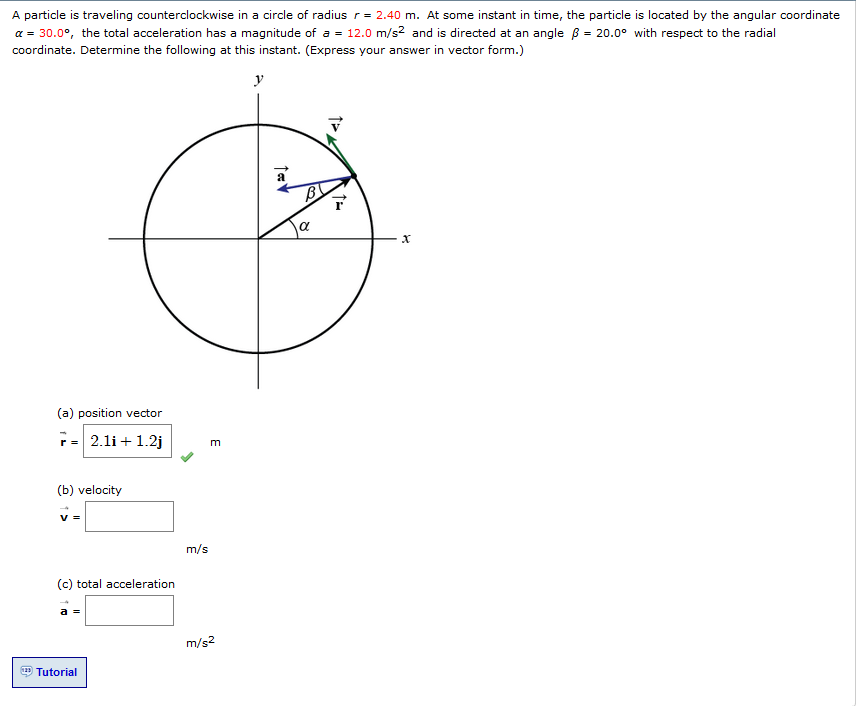A particle is traveling counterclockwise in a circle of radius r= 2.40 m. At some instant in time, the particle is located by the angular coordinate α-30.0°, the total acceleration has a magnitude of a-12.0 m/s2 and is directed at an angle β-20.0o with respect to the radial coordinate. Determine the following at this instant. (Express your answer in vector form.) (a) position vector H 2.11+ 12] (b) velocity 哭: Infss (c) total acceleration Tutorial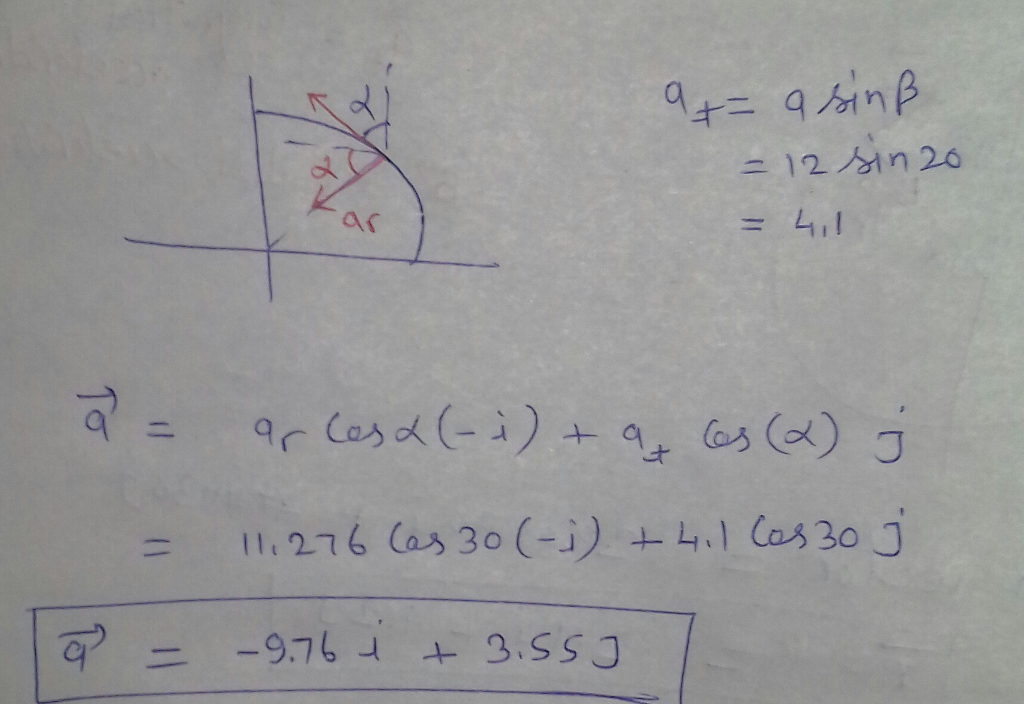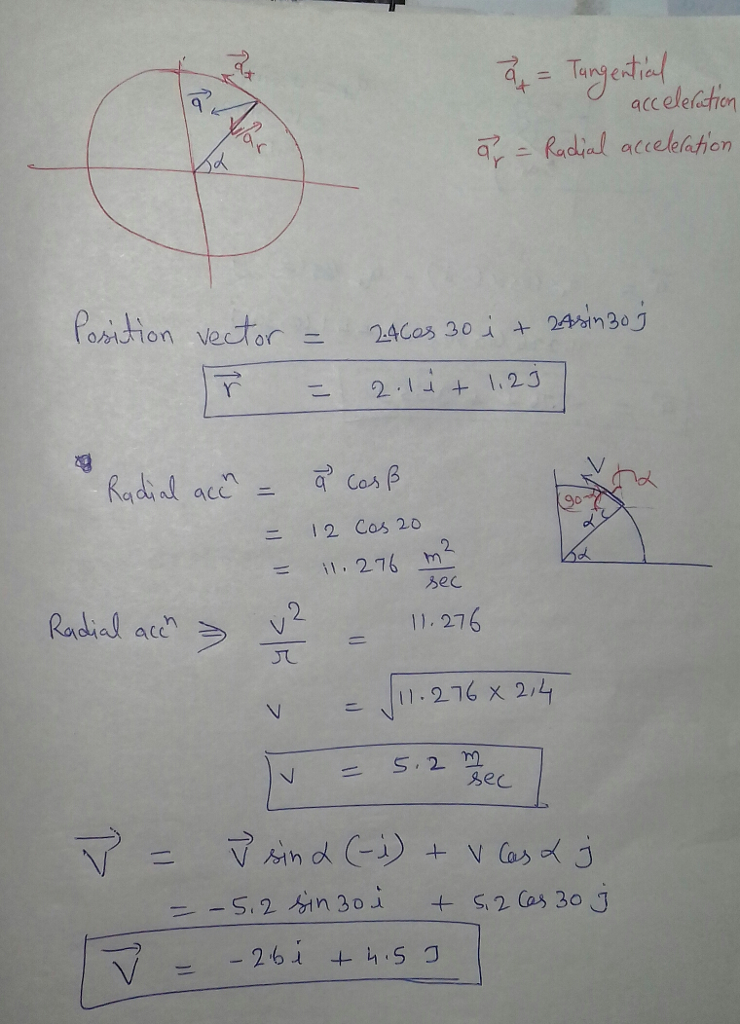#### Earn Coin

Coins can be redeemed for fabulous gifts.

Similar Homework Help Questions
• ### A particle is traveling counterclockwise in a circle of radius r = 2.35 m. At some...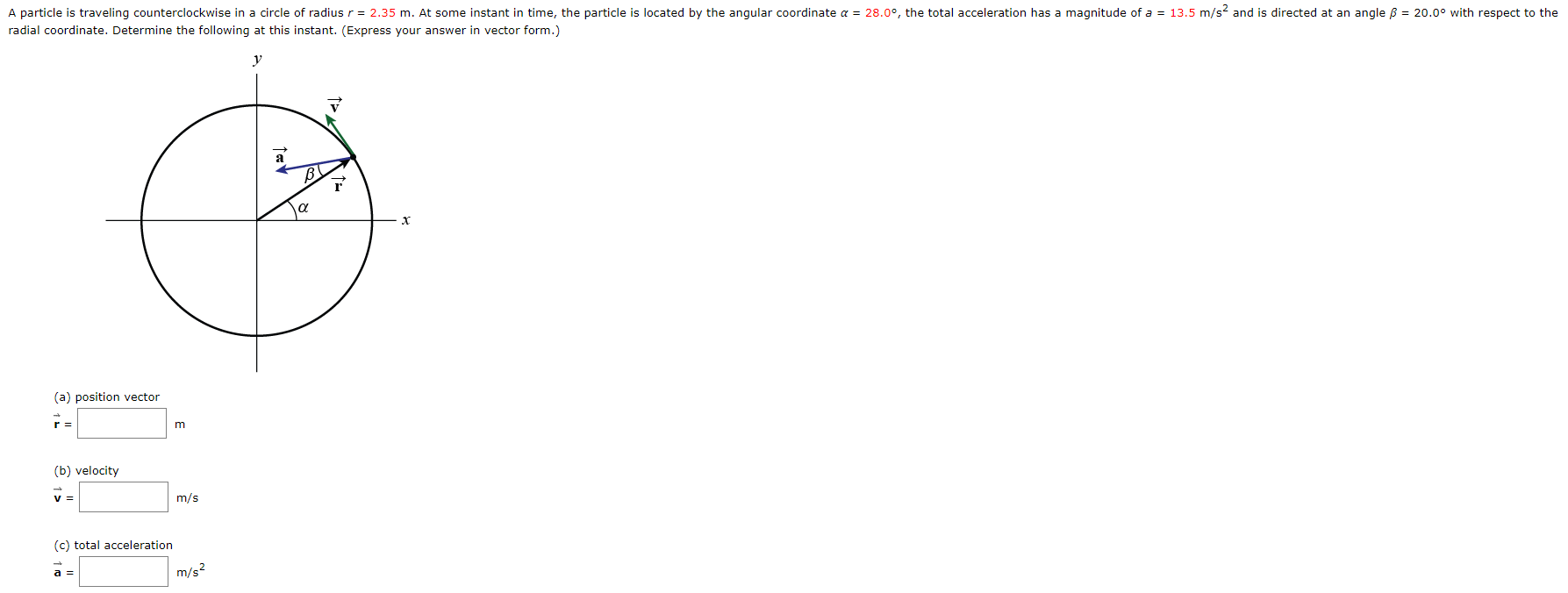A particle is traveling counterclockwise in a circle of radius r = 2.35 m. At some instant in time, the particle is located by the angular coordinate a = 28.0°, the total acceleration has a magnitude of a = 13.5 m/sand is directed at an angle ß = 20.0° with respect to the radial coordinate. Determine the following at this instant. (Express your answer in vector form.) (a) position vector (b) velocity m/s (c) total acceleration

• ### A particle P travels with constant speed on a circle of radius r = 2.40 m...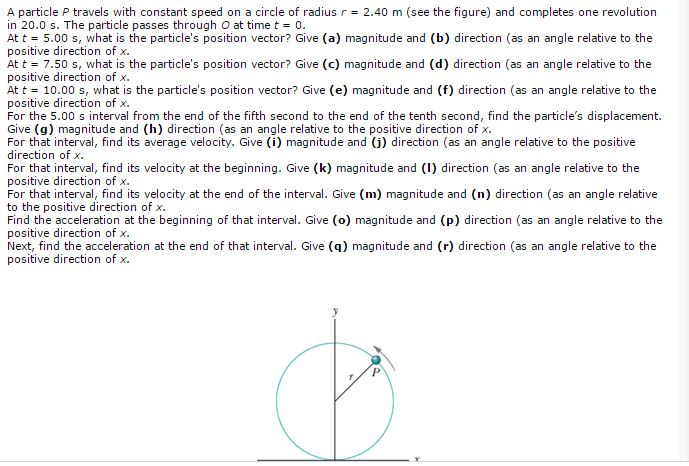A particle P travels with constant speed on a circle of radius r = 2.40 m (see the figure) and completes one revolution in 20.0 s. The particle passes through O at time t = 0. At t = 5.00 s, what is the particle's position vector? Give (a) magnitude and (b) direction (as an angle relative to the positive direction of x. At t = 7.50 s, what is the particle's position vector? Give (c) magnitude and (d) direction...

• ### The disk of radius r = 1.5 m rolls without slipping on the incline with angle...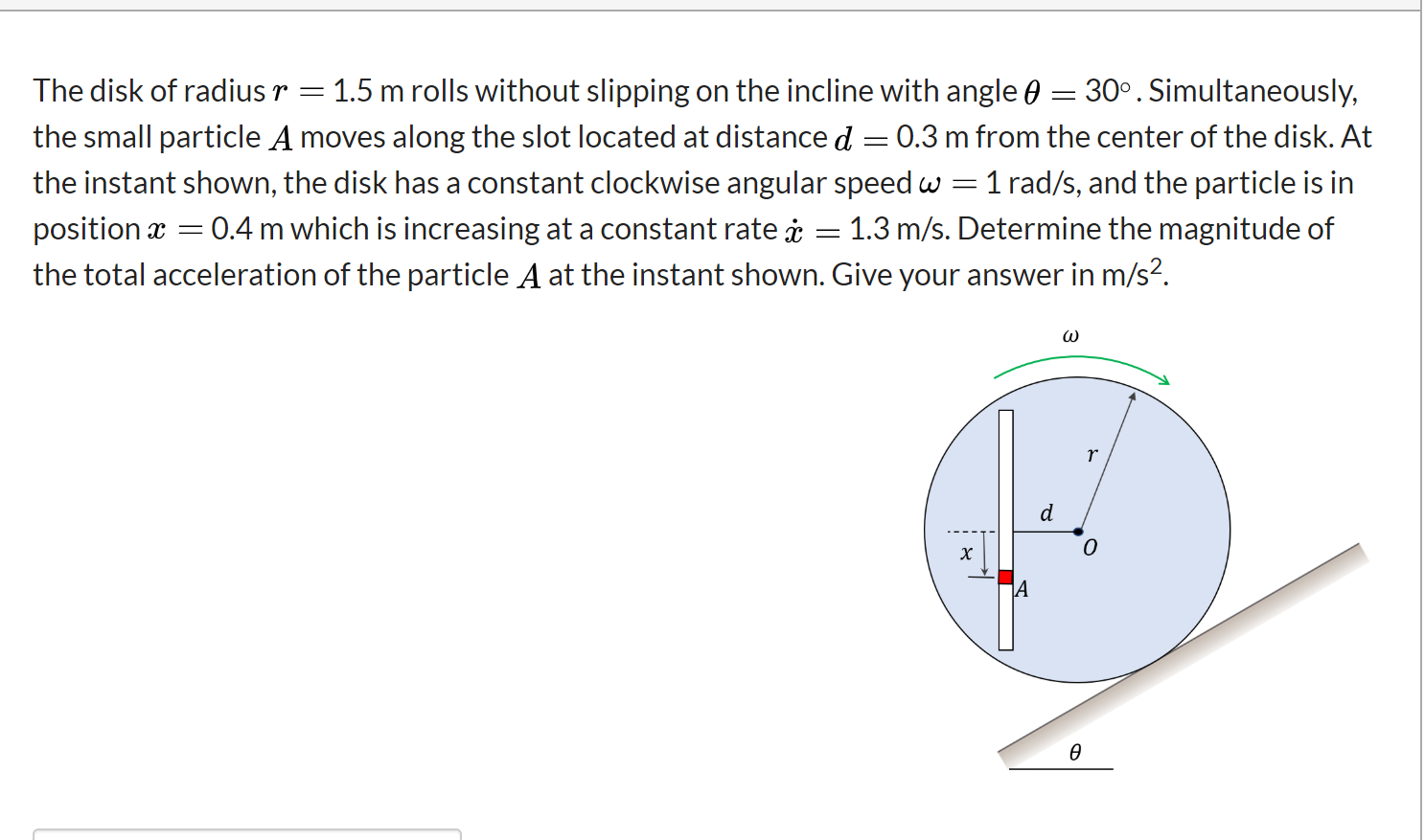The disk of radius r = 1.5 m rolls without slipping on the incline with angle 0 = 30°. Simultaneously, the small particle A moves along the slot located at distance d = 0.3 m from the center of the disk. At the instant shown, the disk has a constant clockwise angular speedw = 1 rad/s, and the particle is in position x = 0.4 m which is increasing at a constant rate į = 1.3 m/s. Determine the magnitude...

• ### b) A particle accelerates around a circle of radius 4 m. At a certain point A,...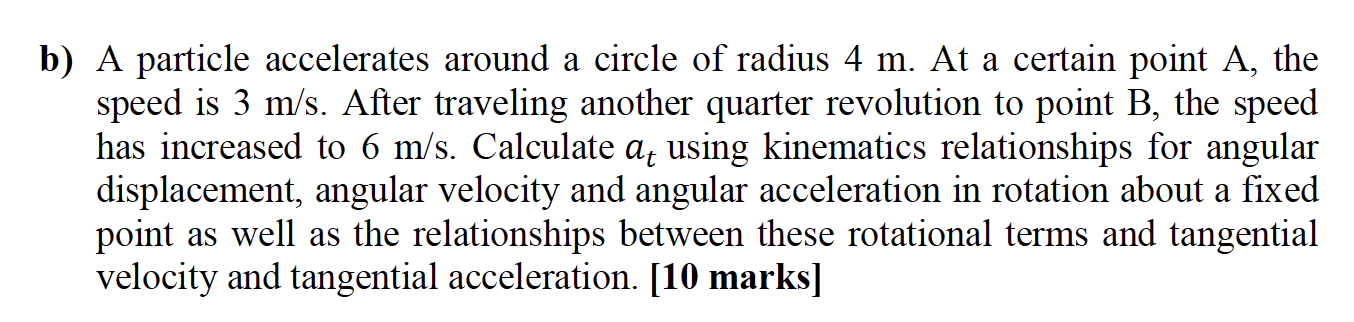b) A particle accelerates around a circle of radius 4 m. At a certain point A, the speed is 3 m/s. After traveling another quarter revolution to point B, the speed has increased to 6 m/s. Calculate at using kinematics relationships for angular displacement, angular velocity and angular acceleration in rotation about a fixed point as well as the relationships between these rotational terms and tangential velocity and tangential acceleration. [10 marks]

• ### At the instant shown, cars A and B are traveling at velocities of 50 m/s and...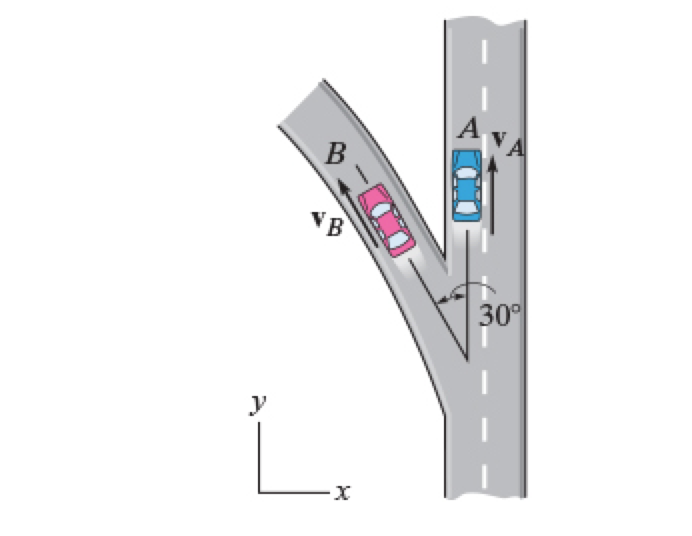At the instant shown, cars A and B are traveling at velocities of 50 m/s and 30 m/s , respectively. B is increasing its velocity by 2 m/s2, while A maintains a constant velocity. The radius of curvature at B is ?B = 200 m. Determine the magnitude of the velocity of B with respect to A. Determine the direction angle of the velocity of B with respect to A. Determine the magnitude of the acceleration of B with respect...

• ### At the instant shown, cars A and B are traveling at velocities of 58 m/s and...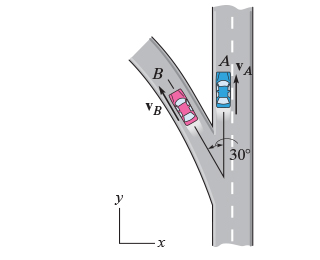At the instant shown, cars A and B are traveling at velocities of 58 m/s and 32 m/s , respectively. B is increasing its velocity by 2 m/s2, while A maintains a constant velocity. The radius of curvature at B is?B = 200 m. Part A Determine the magnitude of the velocity of B with respect to A. Part B Determine the direction angle of the velocity of B with respect to A. Part C Determine the magnitude of the...

• ### A small particle of mass m is at rest on a horizontal circular platform that is...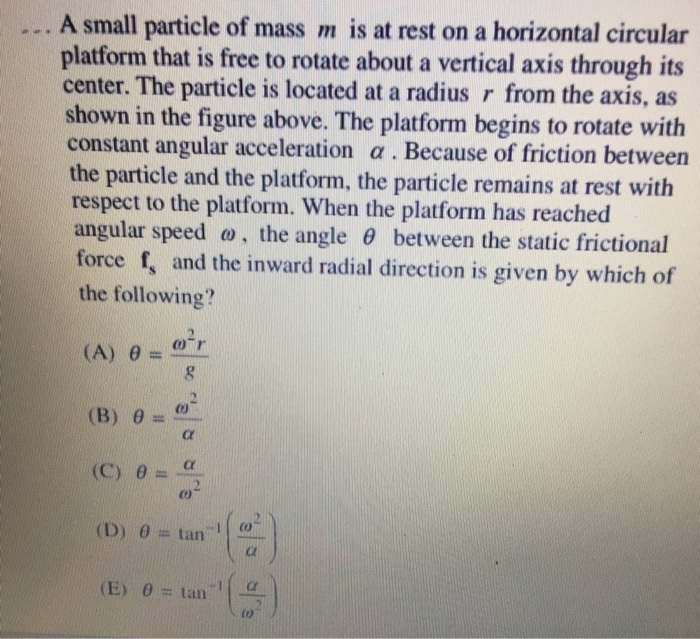A small particle of mass m is at rest on a horizontal circular platform that is free to rotate about a vertical axis through its center. The particle is located at a radius r from the axis, as shown in the figure above. The platform begins to rotate with constant angular acceleration α . Because of friction between the particle and the platform, the particle remains at rest with respect to the platform. When the platform has reached angular speed...

• ### A small object with mass 3.70 kg moves counterclockwise with constant speed 6.10 m/s in a...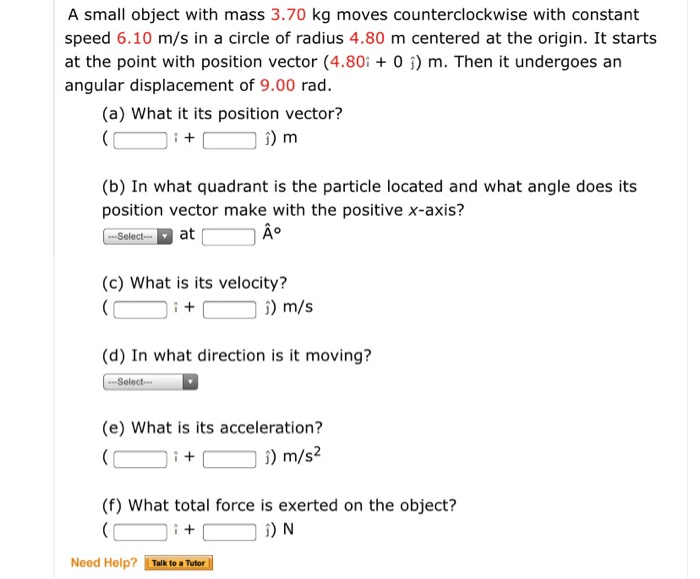A small object with mass 3.70 kg moves counterclockwise with constant speed 6.10 m/s in a circle of radius 4.80 m centered at the origin. It starts at the point with position vector (4.80i 0 ) m. Then it undergoes an angular displacement of 9.00 rad. (a) What it its position vector? 1 + i) m (b) In what quadrant is the particle located and what angle does its position vector make with the positive x-axis? Selectat A° (c) What...

• ### A small object with mass 3.60 kg moves counterclockwise withconstant speed 1.30 rad/s in a...

A small object with mass 3.60 kg moves counterclockwise with constant speed 1.30 rad/s in a circle of radius 3.45 m centered at the origin. It starts at the point with position vector 3.45î m. Then it undergoes an angular displacement of 8.75 rad.(a) What is its new position vector?(b) In what quadrant is the particle located and what angle does its position vector make with the positive x-axis?(c) What is its velocity?(d) In what direction is it moving?(e) What...

• ### A particle of mass m moves in a circle of radius R at a constant speed...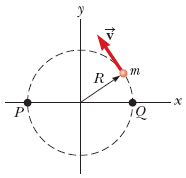A particle of mass m moves in a circle of radius R at a constant speed v, as shown below. The motion begins at point Q at time t = 0. Determine the angular momentum of the particle about the axis perpendicular to the page through point P as a function of time. (Use any variable or symbol stated above along with the following as necessary: t.)